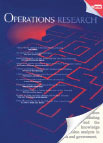# Dynamic Pricing and Learning with Finite Inventories | INFORMSAbstract
We study a dynamic pricing problem with finite inventory and parametric uncertainty on the demand distribution. Products are sold during selling seasons of finite length, and inventory that is unsold at the end of a selling season perishes. The goal of the seller is to determine a pricing strategy that maximizes the expected revenue. Inference on the unknown parameters is made by maximum-likelihood estimation.

We show that this problem satisfies an endogenous learning property, which means that the unknown parameters are learned on the fly if the chosen selling prices are sufficiently close to the optimal ones. We show that a small modification to the certainty equivalent pricing strategy—which always chooses the optimal price w.r.t. current parameter estimates—satisfies Regret(T) = O(log2(T)), where Regret(T) measures the expected cumulative revenue loss w.r.t. a clairvoyant who knows the demand distribution. We complement this upper bound by showing an instance for which the regret of any pricing policy satisfies Ω(log T).

Keywords : marketing: pricing; dynamic programming: Markov, finite state; inventory/production: uncertainty, stochastic